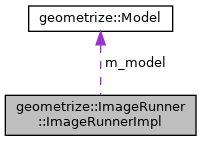Geometrize  1.0 C++ library for geometrizing images into geometric primitives
geometrize::ImageRunner::ImageRunnerImpl Class Reference
Collaboration diagram for geometrize::ImageRunner::ImageRunnerImpl:[legend]

## Public Member Functions

ImageRunnerImpl (const geometrize::Bitmap &targetBitmap)

ImageRunnerImpl (const geometrize::Bitmap &targetBitmap, const geometrize::Bitmap &initialBitmap)

~ImageRunnerImpl ()=default

ImageRunnerImploperator= (const ImageRunnerImpl &)=delete

ImageRunnerImpl (const ImageRunnerImpl &)=delete

std::vector
< geometrize::ShapeResult
step (const geometrize::ImageRunnerOptions &options, std::function< std::shared_ptr< geometrize::Shape >()> shapeCreator, geometrize::core::EnergyFunction energyFunction)

geometrize::BitmapgetCurrent ()

geometrize::BitmapgetTarget ()

const geometrize::BitmapgetCurrent () const

const geometrize::BitmapgetTarget () const

geometrize::ModelgetModel ()

## Private Attributes

geometrize::Model m_model
The model for the primitive optimization/fitting algorithm. More...

## Constructor & Destructor Documentation

 geometrize::ImageRunner::ImageRunnerImpl::ImageRunnerImpl ( const geometrize::Bitmap & targetBitmap )
inline
21 : m_model{targetBitmap} {}
geometrize::Model m_model
The model for the primitive optimization/fitting algorithm.
Definition: imagerunner.cpp:67
 geometrize::ImageRunner::ImageRunnerImpl::ImageRunnerImpl ( const geometrize::Bitmap & targetBitmap, const geometrize::Bitmap & initialBitmap )
inline
22 : m_model{targetBitmap, initialBitmap} {}
geometrize::Model m_model
The model for the primitive optimization/fitting algorithm.
Definition: imagerunner.cpp:67
 geometrize::ImageRunner::ImageRunnerImpl::~ImageRunnerImpl ( )
default
 geometrize::ImageRunner::ImageRunnerImpl::ImageRunnerImpl ( const ImageRunnerImpl & )
delete

## Member Function Documentation

 geometrize::Bitmap& geometrize::ImageRunner::ImageRunnerImpl::getCurrent ( )
inline
42  {
43  return m_model.getCurrent();
44  }
geometrize::Model m_model
The model for the primitive optimization/fitting algorithm.
Definition: imagerunner.cpp:67
geometrize::Bitmap & getCurrent()
getCurrent Gets the current bitmap.
Definition: model.cpp:246

Here is the call graph for this function:const geometrize::Bitmap& geometrize::ImageRunner::ImageRunnerImpl::getCurrent ( ) const
inline
52  {
53  return m_model.getCurrent();
54  }
geometrize::Model m_model
The model for the primitive optimization/fitting algorithm.
Definition: imagerunner.cpp:67
geometrize::Bitmap & getCurrent()
getCurrent Gets the current bitmap.
Definition: model.cpp:246

Here is the call graph for this function:geometrize::Model& geometrize::ImageRunner::ImageRunnerImpl::getModel ( )
inline
62  {
63  return m_model;
64  }
geometrize::Model m_model
The model for the primitive optimization/fitting algorithm.
Definition: imagerunner.cpp:67
 geometrize::Bitmap& geometrize::ImageRunner::ImageRunnerImpl::getTarget ( )
inline
47  {
48  return m_model.getTarget();
49  }
geometrize::Model m_model
The model for the primitive optimization/fitting algorithm.
Definition: imagerunner.cpp:67
geometrize::Bitmap & getTarget()
getTarget Gets the target bitmap.
Definition: model.cpp:241

Here is the call graph for this function:const geometrize::Bitmap& geometrize::ImageRunner::ImageRunnerImpl::getTarget ( ) const
inline
57  {
58  return m_model.getTarget();
59  }
geometrize::Model m_model
The model for the primitive optimization/fitting algorithm.
Definition: imagerunner.cpp:67
geometrize::Bitmap & getTarget()
getTarget Gets the target bitmap.
Definition: model.cpp:241

Here is the call graph for this function:ImageRunnerImpl& geometrize::ImageRunner::ImageRunnerImpl::operator= ( const ImageRunnerImpl & )
delete
 std::vector geometrize::ImageRunner::ImageRunnerImpl::step ( const geometrize::ImageRunnerOptions & options, std::function< std::shared_ptr< geometrize::Shape >()> shapeCreator, geometrize::core::EnergyFunction energyFunction )
inline
28  {
29  const std::int32_t w = m_model.getTarget().getWidth();
30  const std::int32_t h = m_model.getTarget().getHeight();
31  const geometrize::ShapeTypes types = options.shapeTypes;
32
33  if(!shapeCreator) {
34  shapeCreator = geometrize::createDefaultShapeCreator(types, w, h);
35  }
36
37  m_model.setSeed(options.seed);
38  return m_model.step(shapeCreator, options.alpha, options.shapeCount, options.maxShapeMutations, options.maxThreads, energyFunction);
39  }
std::uint32_t getWidth() const
getWidth Gets the width of the bitmap.
Definition: bitmap.cpp:20
geometrize::Model m_model
The model for the primitive optimization/fitting algorithm.
Definition: imagerunner.cpp:67
std::uint32_t shapeCount
The number of candidate shapes that will be tried per model step.
Definition: imagerunneroptions.h:19
ShapeTypes
The ShapeTypes enum specifies the types of shapes that can be used. These can be combined to produce ...
Definition: shapetypes.h:16
geometrize::Bitmap & getTarget()
getTarget Gets the target bitmap.
Definition: model.cpp:241
The maximum number of separate threads for the implementation to use. 0 lets the implementation choos...
Definition: imagerunneroptions.h:22
void setSeed(std::uint32_t seed)
setSeed Sets the seed that the random number generators of this model use. Note that the model also u...
Definition: model.cpp:261
std::function< std::shared_ptr< geometrize::Shape >)> createDefaultShapeCreator(const geometrize::ShapeTypes types, const std::int32_t w, const std::int32_t h)
createDefaultShapeCreator Creates an instance of the default shape creator object. The setup, mutate and rasterize methods are bound with default methods.
Definition: shapefactory.cpp:25
std::vector< geometrize::ShapeResult > step(const std::function< std::shared_ptr< geometrize::Shape >(void)> &shapeCreator, std::uint8_t alpha, std::uint32_t shapeCount, std::uint32_t maxShapeMutations, std::uint32_t maxThreads, const geometrize::core::EnergyFunction &energyFunction=nullptr)
step Steps the primitive optimization/fitting algorithm.
Definition: model.cpp:225
std::uint32_t maxShapeMutations
The maximum number of times each candidate shape will be modified to attempt to find a better fit...
Definition: imagerunneroptions.h:20
std::uint32_t seed
The seed for the random number generators used by the image runner.
Definition: imagerunneroptions.h:21
std::uint8_t alpha
The alpha/opacity of the shapes (0-255).
Definition: imagerunneroptions.h:18
std::uint32_t getHeight() const
getHeight Gets the height of the bitmap.
Definition: bitmap.cpp:25
geometrize::ShapeTypes shapeTypes
The shape types that the image runner shall use.
Definition: imagerunneroptions.h:17

Here is the call graph for this function:## Member Data Documentation

 geometrize::Model geometrize::ImageRunner::ImageRunnerImpl::m_model
private

The model for the primitive optimization/fitting algorithm.

The documentation for this class was generated from the following file:
• /home/travis/build/Tw1ddle/geometrize-lib-docs/geometrize/geometrize/geometrize/runner/imagerunner.cpp